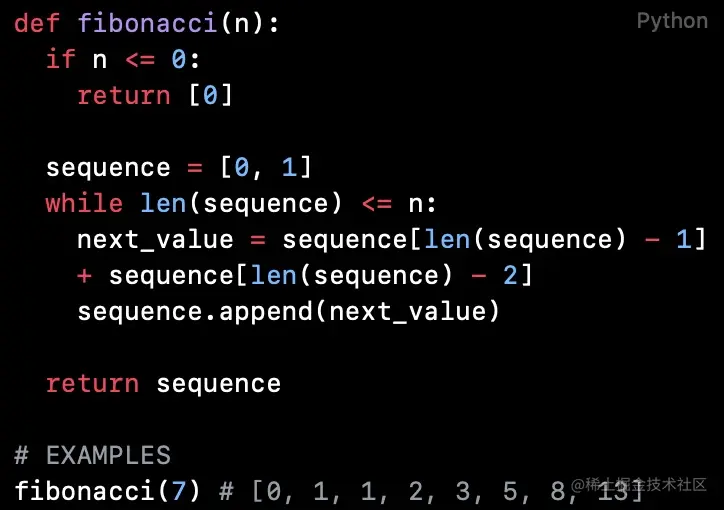# Python code reading (Chapter 54): Fibonacci sequence

2022-01-31 05:36:50 FelixZPython Code reading collection Introduction ： Why not recommend Python Beginners directly look at the project source code

## `fibonacci`

``````def fibonacci(n):
if n <= 0:
return 

sequence = [0, 1]
while len(sequence) <= n:
next_value = sequence[len(sequence) - 1] + sequence[len(sequence) - 2]
sequence.append(next_value)

return sequence

# EXAMPLES
fibonacci(7) # [0, 1, 1, 2, 3, 5, 8, 13]
Copy code ``````

`fibonacci` Function to receive the number of items in the specified Fibonacci sequence `n`, Return the generated Fibonacci sequence .

The function uses a sequence formula and `append()` To generate a sequence .It should be noted that the first term of Fibonacci sequence is `0` term . Fibonacci series formula satisfies the following logic

1. When `n` be equal to `0` when , Function returns the... Of the Fibonacci sequence `0` term ,``.
2. When `n` be equal to `1` when , Function returns the... Of the sequence `0` Xiang and di `1` term ,`[0,1]`.
3. When `n` Greater than or equal to `2` when , Fibonacci Series No `n` Item is by `n-1` and `n-2` The sum of the terms .

According to the function `if` and `while` Judgment logic in , Functions are specifically defined ,`n` Less than `0` When , The function returns ``. `n` Greater than or equal to `2` When , The calculation of Fibonacci sequence by function iteration , Each time the newly calculated item is added to the end of the sequence . Until the calculation of the sequence reaches the `n` term .

form `s.append(x)` Will `x` Add to the end of the sequence , Equate to `s[len(s):len(s)] = [x])`.